# Volume of Prisms and Cylinders Measured in cubic

• Slides: 14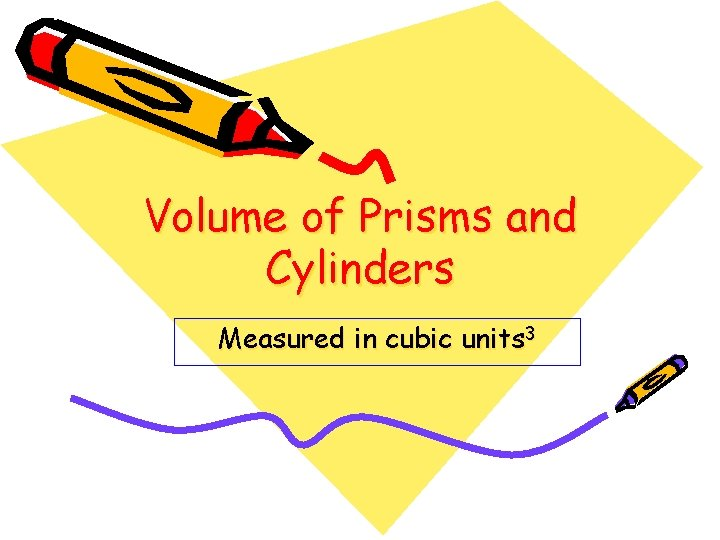Volume of Prisms and Cylinders Measured in cubic units 3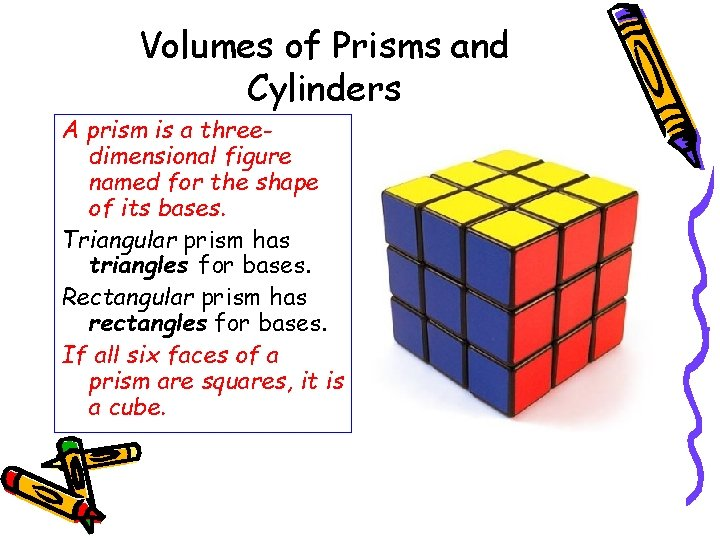Volumes of Prisms and Cylinders A prism is a threedimensional figure named for the shape of its bases. Triangular prism has triangles for bases. Rectangular prism has rectangles for bases. If all six faces of a prism are squares, it is a cube.Triangular prism In this triangular prism the two bases are triangles. The formula for volume of a triangular prism is V = Bh, where B is area of the base and h is height.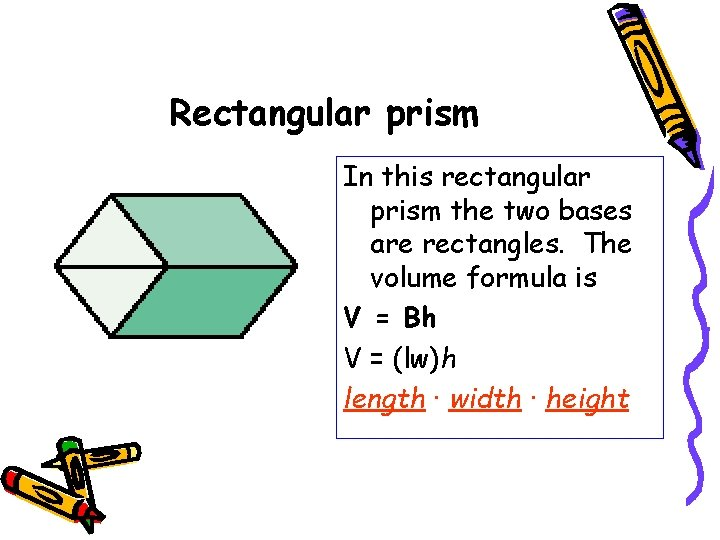Rectangular prism In this rectangular prism the two bases are rectangles. The volume formula is V = Bh V = (lw)h length · width · height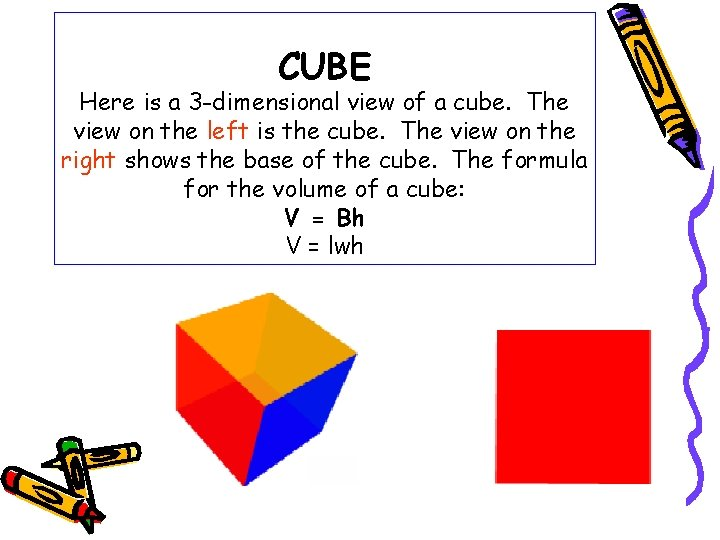CUBE Here is a 3 -dimensional view of a cube. The view on the left is the cube. The view on the right shows the base of the cube. The formula for the volume of a cube: V = Bh V = lwhCylinder: a cylinder is a three-dimensional figure with two circular bases. The volume of a cylinder is the area of the base B times the height h. V = Bh or V = (πr²)h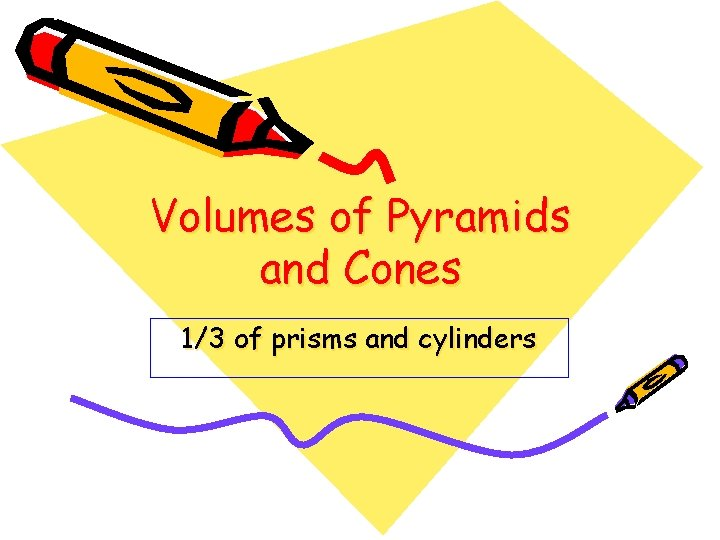Volumes of Pyramids and Cones 1/3 of prisms and cylinders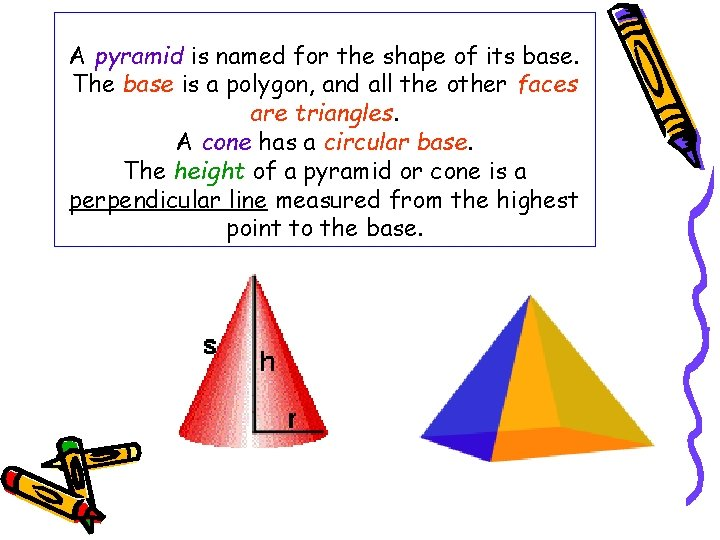A pyramid is named for the shape of its base. The base is a polygon, and all the other faces are triangles. A cone has a circular base. The height of a pyramid or cone is a perpendicular line measured from the highest point to the base.A cone has a circular base. The height of a pyramid or cone is perpendicular line measured from the highest point to the base. In the cone to the left the height is h and the radius of the circular base is r. The s is the slant height which is used to measure surface area of a cone or pyramid. The volume formula for a cone is V = 1/3 Bh or V = 1/3πr²hA pyramid is named for its base. The base is a polygon, and all the other faces are triangles that meet at a common vertex. The height is a perpendicular line from the base to the highest point. The volume formula for a pyramid is V = 1/3 Bh V = 1/3(lw)h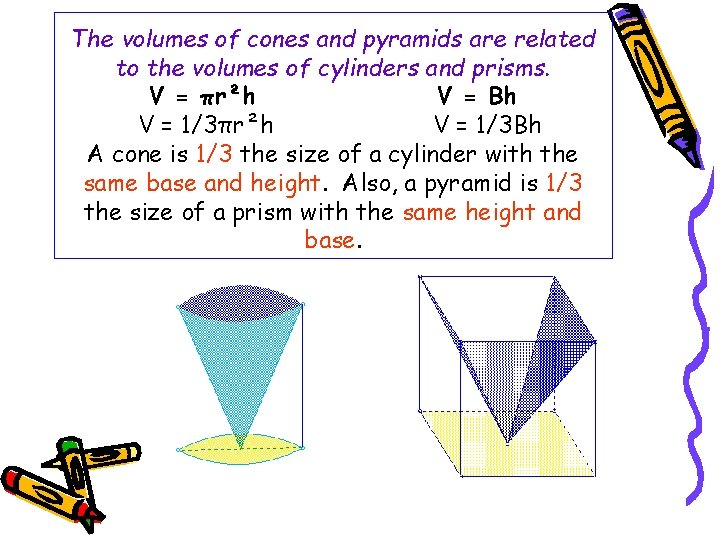The volumes of cones and pyramids are related to the volumes of cylinders and prisms. V = πr²h V = Bh V = 1/3πr²h V = 1/3 Bh A cone is 1/3 the size of a cylinder with the same base and height. Also, a pyramid is 1/3 the size of a prism with the same height and base.Surface Area of Prisms and Cylinders Back to areas 2 againSurface area of objects are used to advertise, inform, create art, and many other things. On the left is an anamorphic image, which is a distorted picture that becomes recognizable when reflected onto a cylindrical mirror.When you flatten-out a three-dimensional object the diagram is called a net. Which of the following answers is the correct net for the cube. Choose a, b, c, or d.